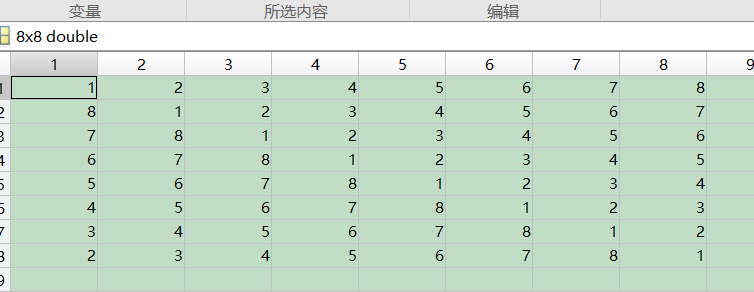• 2021-04-21 09:30:16

我需要循环移动矩阵的各个列.

如果你想将所有列移动相同的数量,这很容易,但是,在我的情况下,我需要将它们全部移动不同的数量.

目前我正在使用循环,如果可能的话,我想删除循环并使用更快的,基于矢量的方法.

我目前的代码

A = randi(2, 4, 2);

B = A;

for i = 1:size( A,2 );

d = randi( size( A,1 ));

B(:,i) = circshift( A(:,i), [d, 0] );

end

是否可以从此代码中删除循环？

更新我测试了所有三种方法,并将它们与本问题中描述的循环进行了比较.我计算了在1000×1000矩阵上按列循环移位100次执行列所需的时间.我重复了几次这个测试.

结果：

>我的循环耗时超过12秒

> Pursuit的建议不到一秒钟

> Zroth的原始答案仅用了2秒多

>安萨里的建议比原始循环慢

最佳答案 编辑

追求是正确的：使用for循环和适当的索引似乎是这里的方式.这是一种方法：

[m, n] = size(A);

D = randi([0, m - 1], [1, n]);

B = zeros(m, n);

for i = (1 : n)

B(:, i) = [A((m - D(i) + 1 : m), i); A((1 : m - D(i) ), i)];

end

原始答案

我以前找过类似的东西,但我从未遇到过一个好的解决方案.使用here之一的算法的修改在我的测试中略微提升了性能：

[m, n] = size(A);

mtxLinearIndices ...

= bsxfun(@plus, ...

mod(bsxfun(@minus, (0 : m - 1)', D), m), ...

(1 : m : m * n));

C = A(idxs);

丑陋？当然.就像我说的那样,它似乎稍快一些(对我来说要快2-3倍);但是对于m = 3000和n = 1000(在相当旧的计算机上),两种算法都在不到一秒的时钟内进行计时.

值得注意的是,对我来说,两种算法似乎都优于Ansari提供的算法,尽管他的回答肯定更直接. (Ansari的算法输出与我的其他两种算法不一致;但这可能只是变换应用的差异.)一般来说,当我尝试使用它时,arrayfun似乎相当慢.细胞阵列对我来说似乎也很慢.但我的测试可能会有所偏见.

更多相关内容
• ## MATLAB循环移位

千次阅读 2021-04-10 08:58:17
matlab循环移位操作 需求，拿到了两个以时间为变量函数，形状差不多但是有时间差，为了比对主要信号区间的差异，需要对齐，便想到了circshift的操作，这个函数感觉做相关，循环卷积这种操作，应该是非常顺手的，...

# matlab循环移位操作

需求，拿到了两个以时间为变量函数，形状差不多但是有时间差，为了比对主要信号区间的差异，需要对齐，便想到了circshift的操作，这个函数感觉做相关，循环卷积这种操作，应该是非常顺手的，记录一下

## 1.circshift用法极其简单

Y = circshift(A,K)

如果指定第几个维度循环位移： Y = circshift(A,K,dim)

K是标量，就对第一维循环位移，K是矩阵，就分别对应的维度循环位移
正数代表下、右方向移位，负数代表左、上方向移位

## 2.举例

% 产生数据------------------------------------
a =

1     2     3
4     5     6
7     8     9
% 向下移位------------------------------------
>> circshift(a,1)
ans =

7     8     9
1     2     3
4     5     6

>> circshift(a,[1,1])
% 向下移位,同时向右移位------------------------
ans =

9     7     8
3     1     2
6     4     5

展开全文• 如给定行向量【1，2，3，4，5，6，7，8】，通过此行向量生成一个循环矩阵如下图， 代码如下： B = [1,2,3,4,5,6,7,8]; BC = zeros(N); for j = 1:N BC(j,:) = circshift(B,[0,j-1]); end

如给定行向量【1，2，3，4，5，6，7，8】，通过此行向量生成一个循环矩阵如下图，代码如下：

B = [1,2,3,4,5,6,7,8];
BC = zeros(N);
for j = 1:N
BC(j,:) = circshift(B,[0,j-1]);
end

展开全文• （1）矩阵循环移位：circshift  例如：b = circshift(a,[x,y]) 其中a为待移位的矩阵，x表示上下移位位数（正数表示向下移位），y表示左右移位位数（正数表示向右移位） >> x = [1,2,3;4,5,6;7,8,9] x = ...

（1）矩阵循环移位：circshift

例如：b = circshift(a,[x,y]) 其中a为待移位的矩阵，x表示上下移位位数（正数表示向下移位），y表示左右移位位数（正数表示向右移位）

>> x = [1,2,3;4,5,6;7,8,9]

x =

1     2     3
4     5     6
7     8     9

>> y = circshift(x,[1,-1])

y =

8     9     7
2     3     1
5     6     4

（2）比特移位：bitshift（不能做循环移位）

例如：b = bitshift(a,k,n) 其中a是待移位数据，k是移位的位数（正数表示左移），n为移位后保留的位数（当左移，超出的位数将被舍弃）

>> x = 131;
>> x_bit = dec2bin(x)

x_bit =

10000011

>> y = dec2bin(bitshift(x,2,8))

y =

1100

要想完成bit的循环移位，可以采用这样的方法：

例如，待移位的数据为x = 3139384450，它是一个32bit的序列，现在需要产生x依此循环左移一位所产生的共32个数据，可以采用以下程序完成:

x = 3139384450;
y = dec2bin(x);     //将10进制变为2进制，这时二进制数据的0，1其实是用字符表示的，这样就形成了一个1x32的行向量

z(1) = x;
for i = 2 : 32
y = circshift(y,[0,-1]);    //对与每一行的数据进行左移,y的行不换,y的前1列置后
z(i) = bin2dec(y);          //将移位后的数据再次变为十进制
end

展开全文• ## MATLAB 数组循环移动

千次阅读 2021-03-03 11:20:57
MATLAB 数组元素循环移动（左移和右移） 在日常使用MATLAB做数组操作时，经常会需要循环位移其中的元素。 网上很多的资料都需要自己编写子函数，其实这大可不必，MATLAB已经内含此函数。 circshift code: A = (1:10)...数组 矩阵 经验分享
• 有一组数列,比如a=[1 2 3 4 5 6 7]，怎样能做出数列[7 1 2 3 4 5 6] ，[6 7 1 2 3 4 5]：   >> a=[1 2 3 4 5 6 7] a =  1 2 3 4 5 6 7   >> a = circshift(a',1)' a =  7
• 该代码使用数组索引来实现矩阵的移位。 它比使用带有“最近邻”... 优化数组索引的灵感来自于一个关于循环移位的片段：Peter J. Acklam ( http://home.online.no/~pjacklam ) 的“MATLAB 数组操作提示和技巧”指南。
• BITROTATE 是内置 BITSHIFT 的一个版本，它执行位模式的循环移位而不是丢弃溢出的位。 在命令提示符下键入“help bitrotate”以获取有关如何使用 BITROTATE 的完整说明。 作为一个很酷的例子，我使用 BITROTATE 将...
• 在这里，我为循环反转和循环移位编写了简单的函数代码。
• 序列移位、非循环移位、可设置移位点数、移位点数政府均可 如有序列：【1 2 36 9 8】， 移位点数：【3】 则输出为：【0 0 0 1 2】；
• matlab循环卷积函数,使用矩阵实现，用于理解循环卷积。分2部分，一部分为循环移位，第二部分给矩阵赋值、计算循环卷积
• 如果一个N点序列在任一方向上移位，那么其结果都不在是位于 0 &lt; = n &lt;= N-1之间。因此，需要进行下面的操作： ...下面给出循环移位的函数： function y = cirshftt(x,m,N) %...
• FCOLSHIFT 在列维度上按向量 s 进行分数循环移位。 y = fcolshift(X, S) 其中 X 是 M 行 x N 列的二维矩阵。 S 是长度为 N 的向量。该函数将矩阵 X 的第 i 列循环移位等于 s[i] 个像素的量。 由 Francois Bouffard ...
• 《利用MATLAB实现循环卷积》由会员分享，可在线阅读，更多相关《利用MATLAB实现循环卷积(8页珍藏版)》请在人人文库网上搜索。1、1、 实验目的1. 利用MATLAB实现循环卷积。2. 比较循环卷积与线性卷积的区别。二、实验...
• Matlab生成循环移位M序列，并计算M序列的自相关1、产生循环移位M序列最近做一个扩频通信方面的东西，需要产生一组扩频码，最简单的就是用一个M序列，循环移位产生过。在用Matlab产生的时候发现Matlab有两个移位命令...
• 按照DFT的频域循环移位定理： 若 ， 则 . 简言之：如果时域序列乘以幂之后再进行DFT，产生的频谱可由的频谱向左移位得到。 我的时域序列是，理论上讲以下方程是成立的 . （1） 方程左右的频谱也应该是相同...
• 一、matlab实现：自定义函数 function text=trans_cipher(strText,operation,key) %%% 对明（密）文采用位移密码进行加（解）密； %%% 明文（密文）:strText （字符串形式！！！）; %%% 操作方式: operation (加密:...
• 一.循环移位相关的协议： 二伪随机序列生成： 伪随机序列在之前的文章中专门讲过，有需要的可以点击下面的文章查看：添加链接描述 三下面是pucch信道生成伪随机序列的matlab代码：
• circshift是循环移位函数。可以使用于数组和矩阵元素的循环移位。 当A是数组 B=circshift(A,p);如果p是正数则实现A从左到右的循环移位。如果p是负数则实现A从右到左的循环移位。 当A是矩阵 B=circshift(A,[p,0])...
• MATLAB_滑动滤波 block name:moving everage 如下图，将random block设置为0~3，周期为0.001的的随机数，sine wave设置成幅值为5,频率为0.5的波形,滑动滤波的遗忘因子分别设置成0~1之间的数值，观察结果有什么差异 ...
• 提到了对序列x(n)做循环移位后的DFT形式为： 上篇博文已经讨论过了第一种实现循环移位的方法，通过在时域中对序列移位，之后取模运算，得到循环移位。并给出了精辟地验证。可以很放心的使用。这篇博文呢？我们就通过...
• ## matlab循环生成矩阵

千次阅读 2021-04-21 06:26:41
MATLAB 的矩阵求值的循环语句?clc;clearall;closeall;t0=;a=[12;34]t=t0;t(1,:)=t0'\an=10;fori=2:nt(i,:)=t(i-1,:)'\a;endtmatlab 中的核矩阵for循环问题A(i,:)-A(j,:)表示A矩阵的第i行减去第j行,得到的是一个...
• %未加循环编译码snrdB_min=-6;snrdB_max=20; %设置信噪比取值的上下限：dB为单位for j=snrdB_min:1:snrdB_maxmax=10000;x=randint(1,max);%随机生成一个二进制序列n=max/4;Fc=20;Fd=10;Fs=40;%Fs为系统的采样频率，...
• 让每个人平竽地捉升口我PAGEPAGE #基于置换移位的单宇节分组加密方法为了减少无线传感器网络编码的冗余字节，提高基于Feistel结构的无线传感器网络分组的 安全性，提出了一种新的单字节分组密码加密方法。...
• 同时，其他人似乎使用向后移位（参见 FEX 22814），因此在使用循环矩阵的人群中显然存在二分法。 我将提供我自己的 circulant.m 版本来涵盖这两种风格。 它允许用户根据需要指定任何一种形式，尽管默认使用向前移位...
• 描述本文主要介绍Matlab如何实现移位寄存器，首先介绍的是移位寄存器的原理及作用，其次介绍了m序列的生成原理及m序列的matlab 仿真实现，最后介绍了Matlab如何实现移位寄存器的代码。移位寄存器的原理及作用1、移位...
• 虽然 circhift 速度很快，并且支持所有类型和输入维度，但它意味着一个循环信号，但情况并非总是如此。 此外，它仅支持整数移位值，而有时需要小数。 参见子图 1 - 天空从地面出来。 有时最好假设图像边界外的零值 ......

# matlab 循环移位matlab 订阅